Ex 12.2

Chapter 12 Class 10 Areas related to Circles (Term 1)
Serial order wise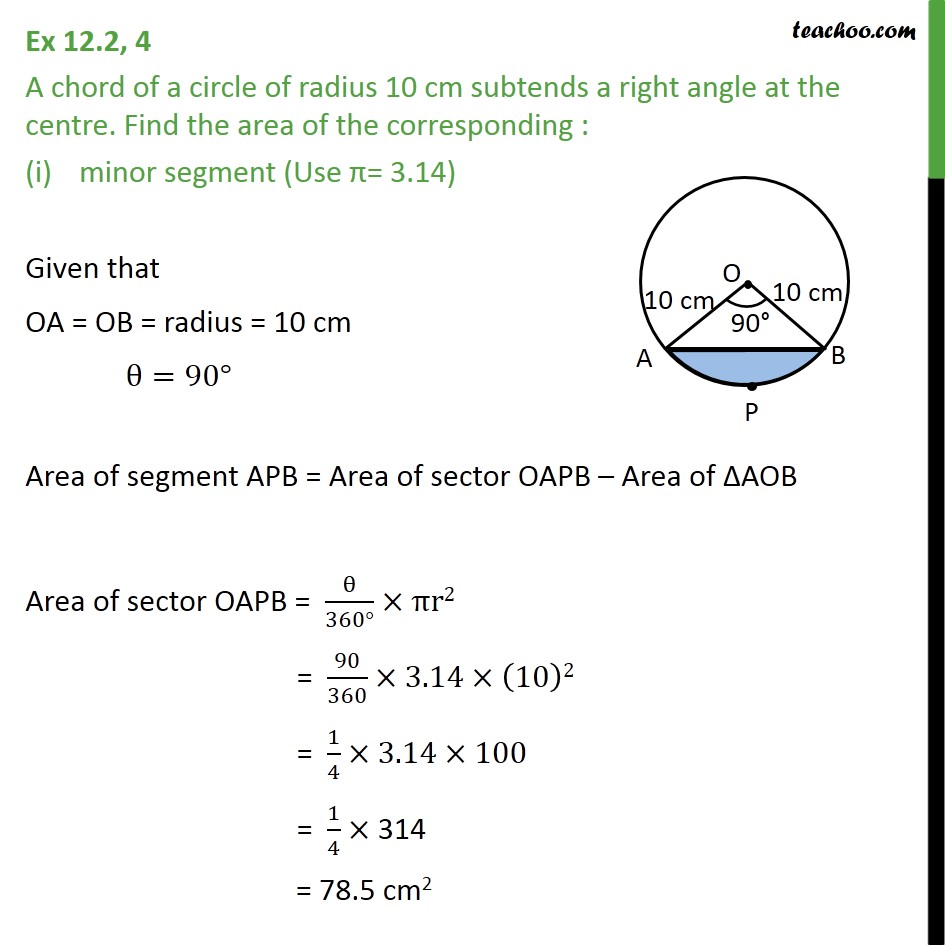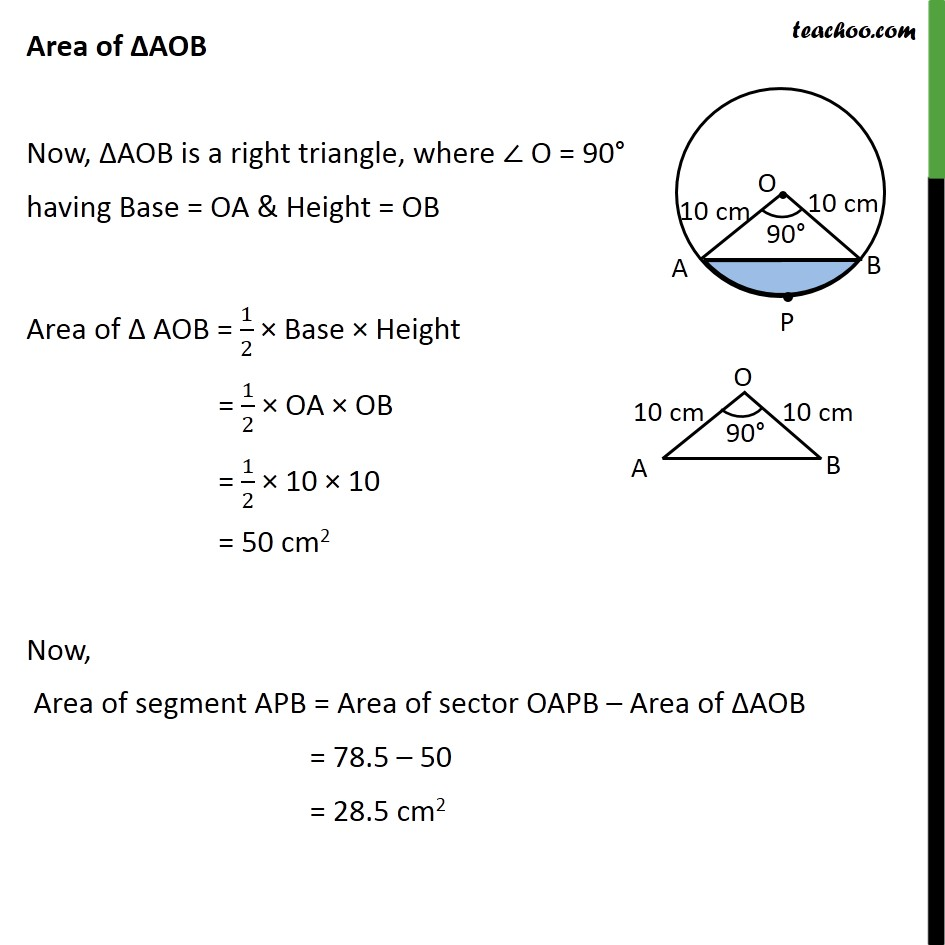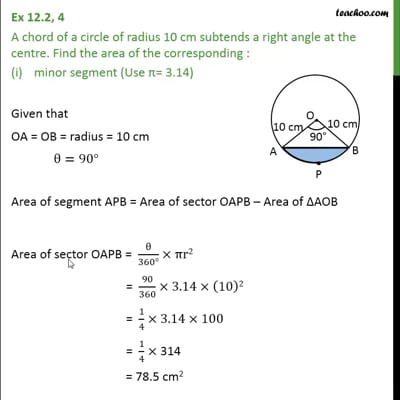This video is only available for Teachoo black users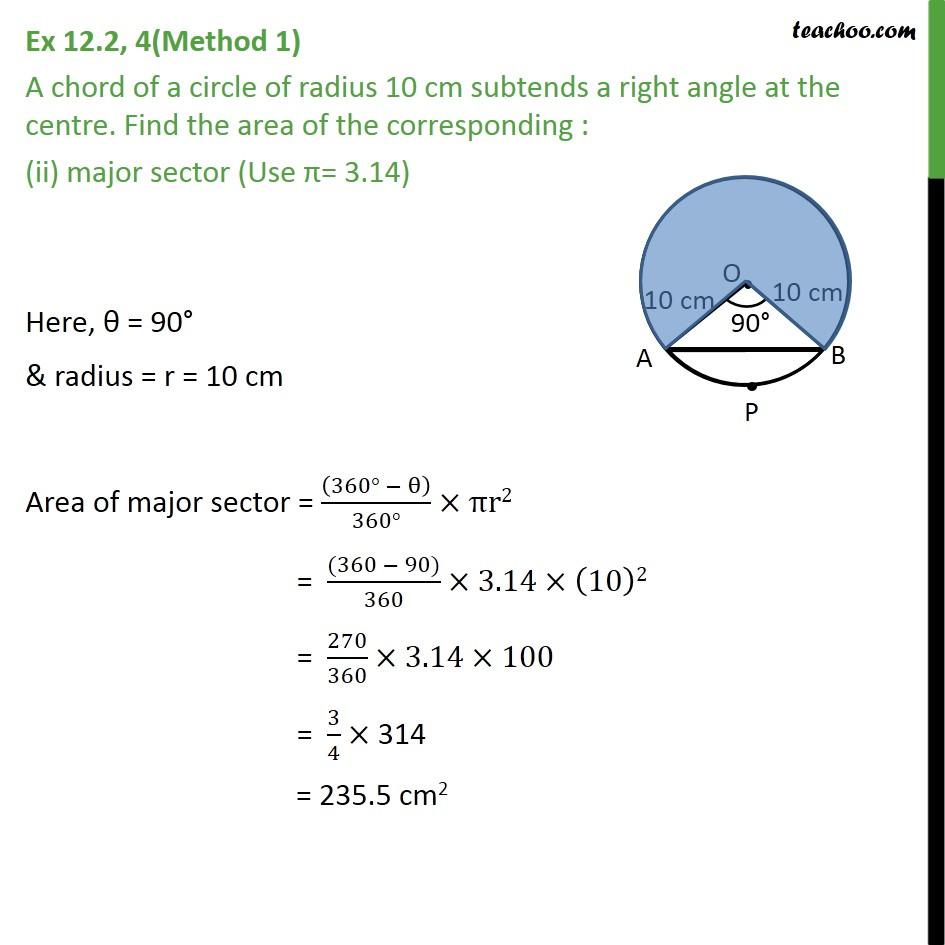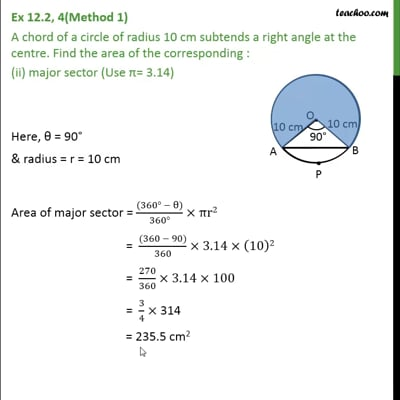This video is only available for Teachoo black users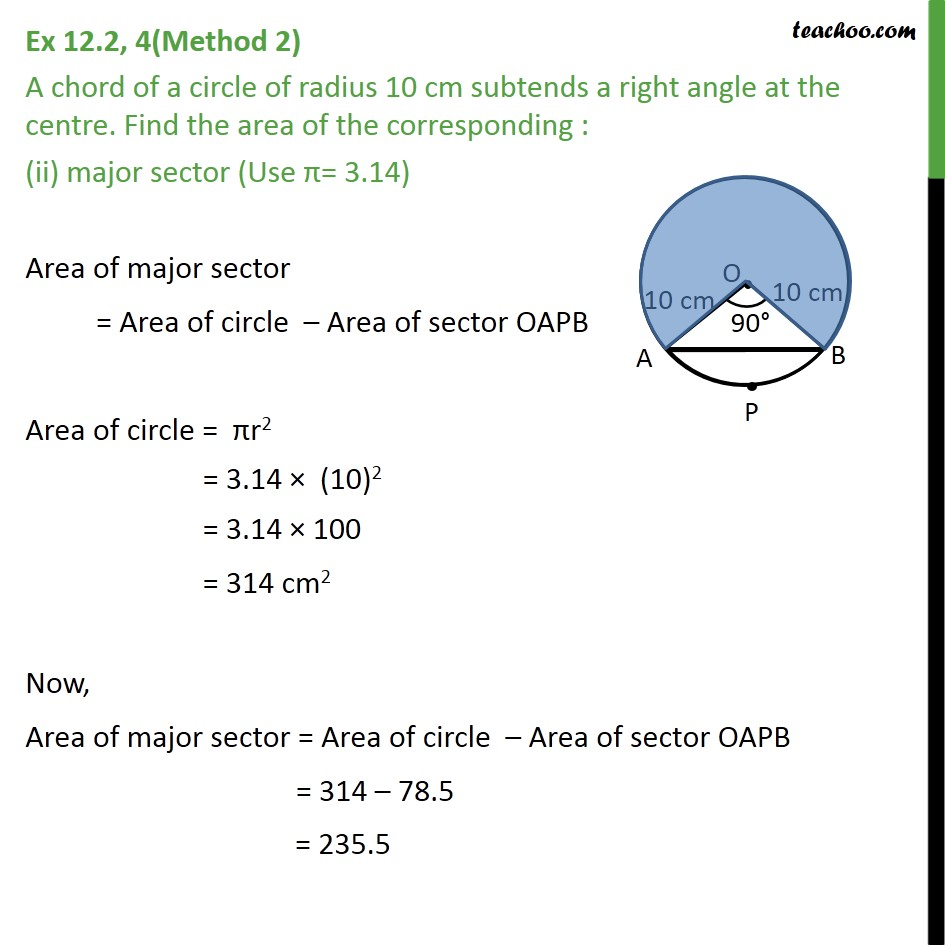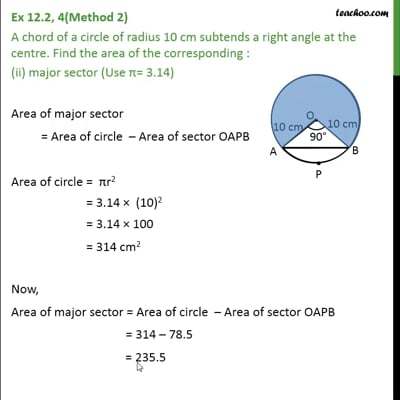This video is only available for Teachoo black users

### Transcript

Ex 12.2, 4 A chord of a circle of radius 10 cm subtends a right angle at the centre. Find the area of the corresponding : minor segment (Use = 3.14) Given that OA = OB = radius = 10 cm =90 Area of segment APB = Area of sector OAPB Area of AOB Area of sector OAPB = /(360 ) r2 = 90/360 3.14 (10)2 = 1/4 3.14 100 = 1/4 314 = 78.5 cm2 Area of AOB Now, AOB is a right triangle, where O = 90 having Base = OA & Height = OB Area of AOB = 1/2 Base Height = 1/2 OA OB = 1/2 10 10 = 50 cm2 Now, Area of segment APB = Area of sector OAPB Area of AOB = 78.5 50 = 28.5 cm2 Ex 12.2, 4(Method 1) A chord of a circle of radius 10 cm subtends a right angle at the centre. Find the area of the corresponding : (ii) major sector (Use = 3.14) Here, = 90 & radius = r = 10 cm Area of major sector = ((360 ))/(360 ) r2 = ((360 90))/360 3.14 (10)2 = 270/360 3.14 100 = 3/4 314 = 235.5 cm2 Ex 12.2, 4(Method 2) A chord of a circle of radius 10 cm subtends a right angle at the centre. Find the area of the corresponding : (ii) major sector (Use = 3.14) Area of major sector = Area of circle Area of sector OAPB Area of circle = r2 = 3.14 (10)2 = 3.14 100 = 314 cm2 Now, Area of major sector = Area of circle Area of sector OAPB = 314 78.5 = 235.5